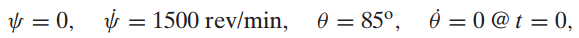### Create an Account

Home / Questions / The system of interest is the fly ball governor in Exercise 741 in the case where the torq...

# The system of interest is the fly ball governor in Exercise 741 in the case where the torque M acting about the vertical axis is zero Suitable generalized coordinates are

The system of interest is the fly ball governor in Exercise 7.41 in the case where the torque M acting about the vertical axis is zero. Suitable generalized coordinates are q1 = ψ and q2 = θ, where ψ is the precession angle. The initial conditions for the system areand the system parameters are m = 250 g, and L = 400 mm.

(a) If properly derived, the Lagrange equation associated with ψ is a perfect differential. The result of integrating that equation is the constant angular momentum about the z axis, which relates the values of ψ˙ and θ corresponding to the specified initial conditions. Solve this equation for ψ˙ at θ = 30°.

(b) Let F have the constant value obtained from the Lagrange equation when θ = 30o, θ˙ ≡ 0, and ψ˙ has the value found in Part (a). Use numerical methods to solve the equations of motion corresponding to this value of F and the specified initial conditions. Plot ψ and θ as functions of t for an interval sufficiently long to ascertain whether θ = 30o is a dynamic equilibrium state. Check the correctness of the integration by monitoring whether the angular momentum about the vertical axis is constant. (c) An alternative value of F is found by simultaneously solving the angular momentum equation in Part (a) and the work–energy principle ΔT + ΔV = FΔyB, where ΔyB is the upward displacement of the vertical bar to which F is applied. The initial conditions for this evaluation of F are those specified and θ = 30° and θ˙ = 0 at the second position. The value of F obtained in this manner does the minimum amount of work required to attain the second state, but it is possible that this value of F would not be adequate to move the system beyond some intermediate position. Solve the equations of motion with the initial conditions set at the specified values and F equated to this minimumwork value. Does the system actually arrive at θ = 30° with θ˙ = 0?

Jun 16 2020 View more View LessSubscribe To Get Solution